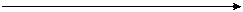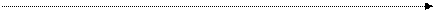# THE MEANING OF GEOMETRY.Geometry is a very old subject. It probably began in Babilonia and Egypt. Men needed practical ways for measuring their land, for building pyramids, and for defining volumes. The Egyptians were mostly concerned with applying geometry in their everyday problems. Yet, as the knowledge of the Egyptians spread to Greece the Greeks found the ideas about geometry very intriguing and misterious. The Greeks began to ask “Why? Why is that true?” In 300 B. C. all the known facts about Greek geometry were put into a logical sequence by Euclid. His book, called Elements,. is one of the most famous books of mathematics. In recent years men have improved on Euclid’s work. Today geometry includes not only the study of the shape and size of the earth and all things on it, but also the study of relations between geometric objects. The most fundamental idea in the study of geometry is the idea of a point. We will not try to define what a point is, but instead discuss some of its properties. Think of a point as an exact location in space. You cannot see a point, feel a point, or move a point, because it has no dimensions. There are points (locations) on the earth, in the earth, in the sky, on the sun, and everywhere in space. When writing about points, you represent the points by dots. Remember the dot is only a picture of a point and not the point itself.

Points are commonly referred to by using capital letters. The dots below mark points and are referred to as point A, point B, and point C.

C

.

A

.

B

.

If you mark two points on your paper and, by using a ruler, draw a straight line between them, you will get a figure. The figure below is a picture of a line segment.DE

Points D and E are referred to as endpoints of the line segment. The line segment includes point D, point E, and all the points between them. Imagine extending the segment indefinitely. It is impossible to draw the complete picture of such an extension but it can be represented as follows.D E

Let us agree on using the word line to mean a straight line. The figure above is a picture of line DE or line ED.

Points and Lines

The world around us contains many physical objects from which mathematicians have developed geometric ideas. These objects can serve as models of the geometric figures. The edge of a ruler, or an angle of this page is a model of a line. We have agreed to use the word line to mean a straight line. A geometric line is the property these models of lines have in common; it has length but no thickness and no width; it is an idea. A particle of dust in the air, or a dot on a piece of paper is a model of a point. A point is an idea about an exact location; it has no dimensions. We usually use letters of the alphabet to name geometric ideas. For example, we speak of the following models of points as point A, point B, and point C.

. B

. A

. C

We speak of the following as line AB or line BA.

A BThe arrows on the model above indicate that a line extends indefinitely in both directions. Let us agree to use the symbol “arrow” to mean a line. Line AB means line AB. Can you locate a point C between A and B on the drawing of the line AB above? Could you locate another point between B and C? Could you continue this process indefinitely? Why? Because between two points on a line there is another point. A line consists of a set of points. Therefore a piece of a line is a subset of the line. There are many kinds of subsets of a line. The subset (piece) of the arrow AB shown below is called a line segment. The symbol for the line segment AB is line AB. Points A and B are the endpoints, as you may remember. A line segment is a set of points consisting of the two endpoints and all of the points on the line between them. How does a line segment differ from a line? Could you measure the length of a line? Of a line segment? A line segment has definite length but a line extends indefinitely in each of its directions. Another important subset of a line is called a ray. That part of the line MN shown below is called ray NM. The symbol for ray MN is ray MN.N

M

A ray has indefinite length and only one endpoint. The endpoint of a ray is called its vertex. The vertex of the ray MN is M. In the drawings above you see pictures of a line, a line segment, and a ray – not the geometric ideas they represent. Let us agree that to draw a geometric figure means to draw its picture

Дата добавления: 2015-12-01; просмотров: 1595; ЗАКАЗАТЬ НАПИСАНИЕ РАБОТЫ

Поиск по сайту:

При помощи поиска вы сможете найти нужную вам информацию, введите в поисковое поле ключевые слова и изучайте нужную вам информацию.

Поделитесь с друзьями:

Если вам понравился данный ресурс вы можете рассказать о нем друзьям. Сделать это можно через соц. кнопки выше.
helpiks.org - Хелпикс.Орг - 2014-2020 год. Материал сайта представляется для ознакомительного и учебного использования. | Поддержка
Генерация страницы за: 0.016 сек.